Next: About this document ... Up: lab_template Previous: lab_template

Subsections

# Parametric Curves in the Plane

## Purpose

The purpose of this lab is to introduce you to curve computations using Maple for parametric curves and vector-valued functions in the plane.

## Background

By parametric curve in the plane, we mean a pair of equations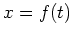and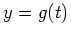for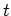in some interval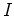. A vector-valued function in the plane is a function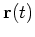that associates a vector in the plane with each value ofin its domain. Such a vector valued function can always be written in component form as follows,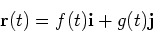whereand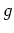are functions defined on some interval. From our definition of a parametric curve, it should be clear that you can always associate a parametric curve with a vector-valued function by just considering the curve traced out by the head of the vector.

## Plotting and animating curves in the plane

The ParamPlot command is in the CalcP package so you have to load it first. If you get an error from this command, ask for help right away.
>with(CalcP7);

The ParamPlot command produces an animated plot. To see the animation, execute the command and then click on the plot region below to make the controls appear in the Context Bar just above the worksheet window.
>ParamPlot([t,t^2],t=-2..2);

the direction of the motion on the curve can be reversed by simply changing the first component from t to -t, as shown below.
>ParamPlot([-t,t^2],t=-2..2);

The ParamPlot command is nice for visualization, but its output doesn't always show up in printouts. Toproduce a printable plot, you can use the VPlot command as shown below.
>VPlot([t^2,t^3-t],t=-1.5..1.5);


## Defining parametric curves and vector valued functions simply in Maple

The easiest way to define a vector function or a parametric curve is to use the Maple list notaion with square brackets[]. Strictly speaking, this does not define something that Maple recognizes as a vector, but it will work with all of the commands you need for this lab.
>f:=t->[2*cos(t),2*sin(t)];

You can evaluate this function at any value of t in the usual way.
>f(0);

This is how to access a single component. You would use f(t) to get the second component.
>f(t)


## Exercises

1. Consider the simple function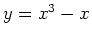. Animate the following two parameterizations for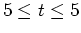and state how the two parameterizations of the same function are different.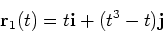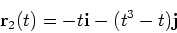2. Consider the curvefor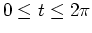. Plot the graph ofand calculate the points atand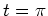. Then plot the points on the graph.

3. Suppose that at time zero, flight 12 is at the point (100 mi, -101.63347 mi) at an altitude of 30,000 feet and traveling northwest at 429 mph and that flight 33 is at the same altitude, but is traveling due east at a speed of 388 mph. At time zero, flight 33 is at the point (-200 mi, 30 mi).
1. Write a parametric function for each flight.
2. Animate the flights on one plot.
3. Do the planes crash? When(convert your answer to minutes)?Next: About this document ... Up: lab_template Previous: lab_template
Jane E Bouchard
2007-09-10